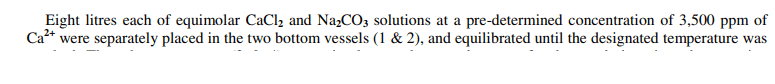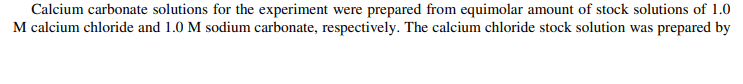# Determining the concentration of ions in ppm

• Chemistry
rwooduk
Homework Statement:
It's in a paper I am reading (not homework)
Relevant Equations:
ppm = (mass of solute ÷ mass of solution x 1,000,000
I'm a little confused by a basic statement in a paper I am reading. It says that calcium chloride (1 M) and sodium carbonate (1 M) i.e. equimolar, have "a predetermined concentration of 3500 ppm of Ca2+"...I understand that the mixing of these two will cause a precipitation reaction, but don't understand how the ppm of calcium ions was determined, was it measured / calculated somehow? Why would there be Ca2+ in the sodium carbonate solution? The statement seems a little confusing.

Thank you in advance for any help understanding this.

EDIT paper is Calcium carbonate scale formation in pipes: effect of flow rates, temperature, and malic acid as additives on the mass and morphology of the scale

Homework Helper
It says they are equimolar; that is the molar concentrations of sodium carbonate and calcium chloride are equal to each other; they are not necessarily equal to 1M. 3500ppm Ca2+ is 0.0875M. There is no Ca in the sodium carbonate solution - there can't be; the statement must mean that the (equal) molar concentration was chosen to give 3500ppm Ca2+ in the calcium chloride solution. It is a little awkwardly expressed, I agree.

•rwooduk
rwooduk
It says they are equimolar; that is the molar concentrations of sodium carbonate and calcium chloride are equal to each other; they are not necessarily equal to 1M. 3500ppm Ca2+ is 0.0875M. There is no Ca in the sodium carbonate solution - there can't be; the statement must mean that the (equal) molar concentration was chosen to give 3500ppm Ca2+ in the calcium chloride solution. It is a little awkwardly expressed, I agree.

Thank you mjc. So, then I guess that the ppm was calculated by using the mass of calcium chloride required to give 1 M solution divided by the mass of solution, would that be correct?

Also, it says...So, that would be equimolar right?

Homework Helper
So, then I guess that the ppm was calculated by using the mass of calcium chloride required to give 1 M solution divided by the mass of solution, would that be correct?
No. First, it is ppm of calcium, not calcium chloride. Second, I have already told you that 3500 ppm Ca corresponds to a molarity of 0.0875M, not 1M.
So, that would be equimolar right?
Yes. You didn't tell us this before. Is there any more information you're hiding? It's not clear to me now what's going on, or what the 3500ppm refers to. It can't be the stock solutions if they are 1M. Are the stock solutions diluted before being mixed? Your quote suggests not. Is 3500ppm the concentration of Ca2+ after mixing the solutions? I wouldn't have thought so; CaCO3 is not that soluble. I'm really confused now.

Mentor

•jim mcnamara
rwooduk
Apologies for the confusion and thank you for the further help with this. The paper is Calcium carbonate scale formation in pipes: effect of flow rates, temperature, and malic acid as additives on the mass and morphology of the scale

The excerpts from the paper which I uploaded as images are from Section 2.1.

https://www.sciencedirect.com/science/article/pii/S1876619614000102

Homework Helper
Hmmm. It's still not quite clear. It looks like 3500 ppm is the concentration of Ca2+ in the mixed solution. The initial concentration would be 0.5M (20,000 ppm), and I would expect the concentration after complete precipitation to be lower than 3500, but this is a flow system, and deposition is slow, so maybe 3500 ppm is a dynamic steady-state value. They could have been clearer. (For example, the wording literally implies that the sodium carbonate solution was made from calcium chloride crystals!)

•rwooduk
rwooduk
Hmmm. It's still not quite clear. It looks like 3500 ppm is the concentration of Ca2+ in the mixed solution. The initial concentration would be 0.5M (20,000 ppm), and I would expect the concentration after complete precipitation to be lower than 3500, but this is a flow system, and deposition is slow, so maybe 3500 ppm is a dynamic steady-state value. They could have been clearer. (For example, the wording literally implies that the sodium carbonate solution was made from calcium chloride crystals!)

Thanks mjc for taking the time to read the section of the paper. Yes, I was quite confused by their description. Your interpretation has been a great help. Thank you.

•